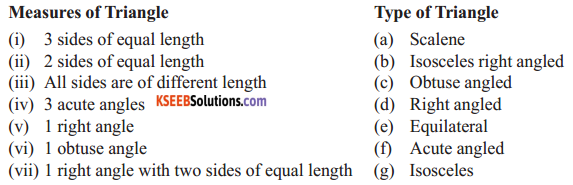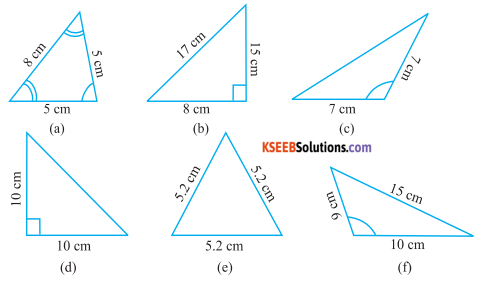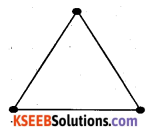# KSEEB Solutions for Class 6 Maths Chapter 5 Understanding Elementary Shapes Ex 5.6

Students can Download Chapter 5 Understanding Elementary Shapes Ex 5.6 Questions and Answers, Notes Pdf, KSEEB Solutions for Class 6 Maths helps you to revise the complete Karnataka State Board Syllabus and score more marks in your examinations.

## Karnataka State Syllabus Class 6 Maths Chapter 5 Understanding Elementary Shapes Ex 5.6

Question 1.
Name the types of following triangles:
Solution:
a) Triangle with lengths of sides 7 cm,8 cm, and 9 cm.
Scalene triangle

b) ∆ ABC with AB = 8.7 cm, AC = 7 cm, and BC = 6 cm.
Scalene trianglec) ∆ PQR such that PQ = QR = PR = 5 cm.
Equilateral triangle

d) ∆ DEE with m∠D = 90°
Right – angled triangle

e) ∆ XYZ with m∠Y = 90°and XY = YZ
Right – angled isosceles triangle

f) ∆ LMN with m∠L = 30°, m∠M = 70° and m∠N = 80°
Acute angled triangle.Question 2.
Match the following :Solution:
1 – (e),
2 – (g),
3 – (a),
4 – (f),
5 – (d),
6 – (c),
7 – (b).

Question 3.
Name each of the following triangles in two different ways : (You may judge the nature of the angle by observation)
Solution:a) Acute angle and isosceles.
b) Right – angle and scalene
c) Obtuse – angled and isosceles
d) Act Right – angled and isosceles
e) Acute – angles and equilateral
f) Obtuse angled and scalene.Question 4.
Try to construct triangles using match sticks, some are shown here. Can you make a triangle witha) 3 matchsticks?
b) 4 matchsticks?
c) 5 matchsticks?
d) 6 matchsticks?
(Remember you have to use all the available matchsticks in each case)
Name the type of triangle in each case. If you cannot make a triangle, think of reasons for it.
Solution:
a) By using 3 matchsticks. we can from as triangle asb) By using 4 matchsticks, we cannot form a triangle, of this is because the sum of the lengths
of any two sides of a triangle is always greater than the length of the remaining side of the triangle.
c) By using 5 matchsticks, we can form a triangle as.d) By using 6 matchsticks, we can from a triangle as.